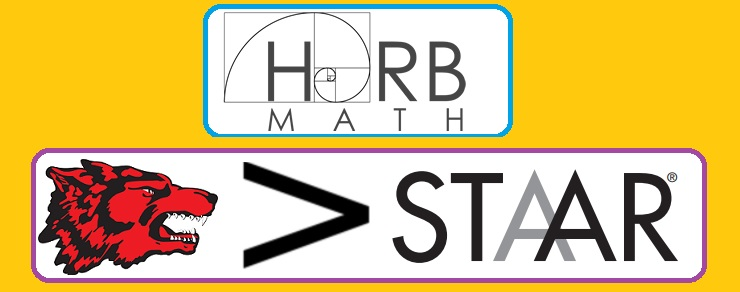# ⛧ Q 43 GRT : Quadratic equation vertex form given vertex and a point⛧

10 Questions
50 Minutes
⛧ Q 43 - STAAR 2017⛧
GRT : Quadratic equation vertex form given vertex and a point

School ID